# Electric Forces and Electric Fields Physics Static Electric

• Slides: 63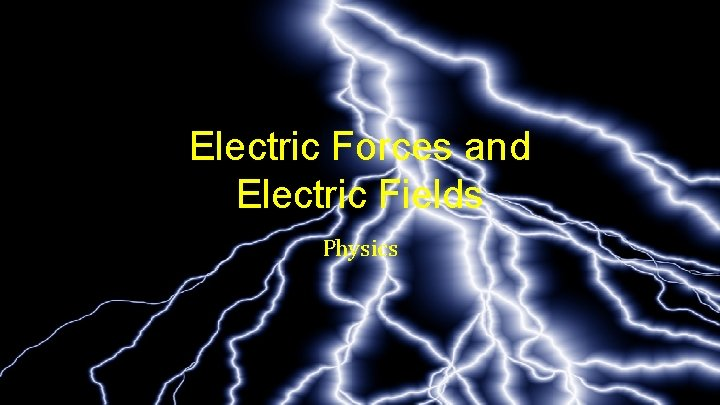Electric Forces and Electric Fields PhysicsStatic Electric Charge and Conductors •Static Electric Charge and Conductors •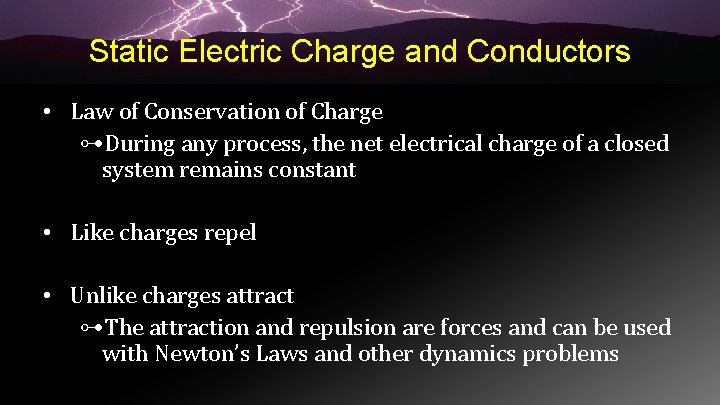Static Electric Charge and Conductors • Law of Conservation of Charge ⊶During any process, the net electrical charge of a closed system remains constant • Like charges repel • Unlike charges attract ⊶The attraction and repulsion are forces and can be used with Newton’s Laws and other dynamics problems08 -01 Static Electric Charge and Conductors Electric Ink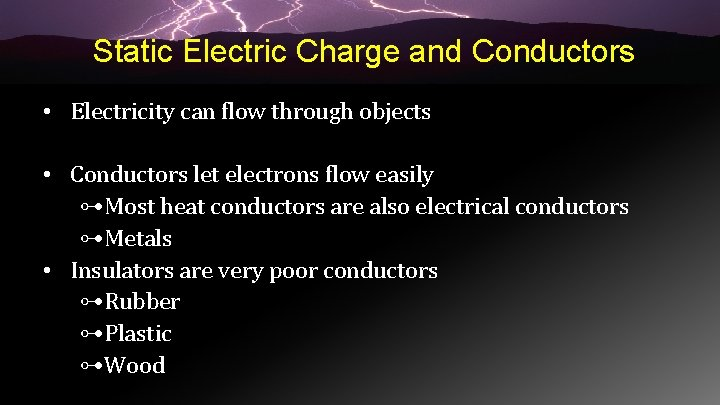Static Electric Charge and Conductors • Electricity can flow through objects • Conductors let electrons flow easily ⊶Most heat conductors are also electrical conductors ⊶Metals • Insulators are very poor conductors ⊶Rubber ⊶Plastic ⊶Wood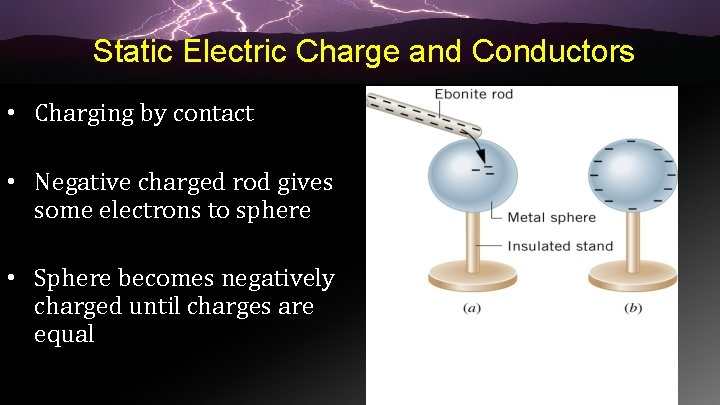Static Electric Charge and Conductors • Charging by contact • Negative charged rod gives some electrons to sphere • Sphere becomes negatively charged until charges are equal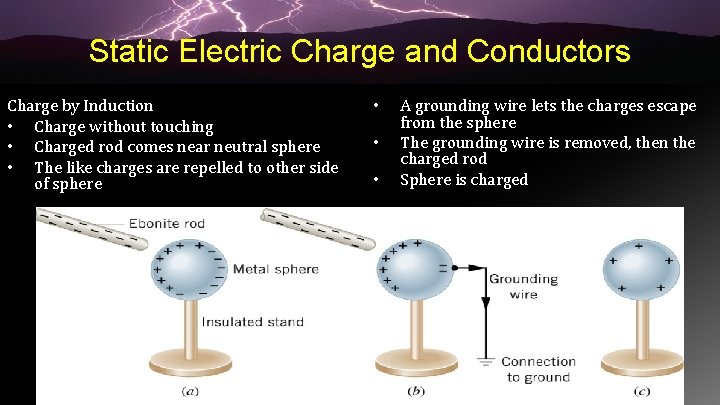Static Electric Charge and Conductors Charge by Induction • Charge without touching • Charged rod comes near neutral sphere • The like charges are repelled to other side of sphere • • • A grounding wire lets the charges escape from the sphere The grounding wire is removed, then the charged rod Sphere is charged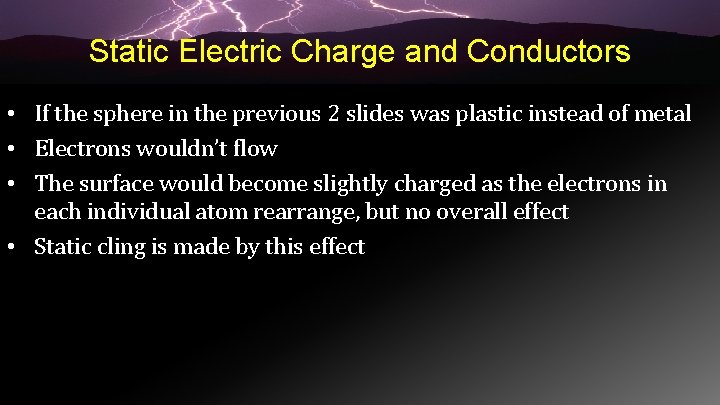Static Electric Charge and Conductors • If the sphere in the previous 2 slides was plastic instead of metal • Electrons wouldn’t flow • The surface would become slightly charged as the electrons in each individual atom rearrange, but no overall effect • Static cling is made by this effect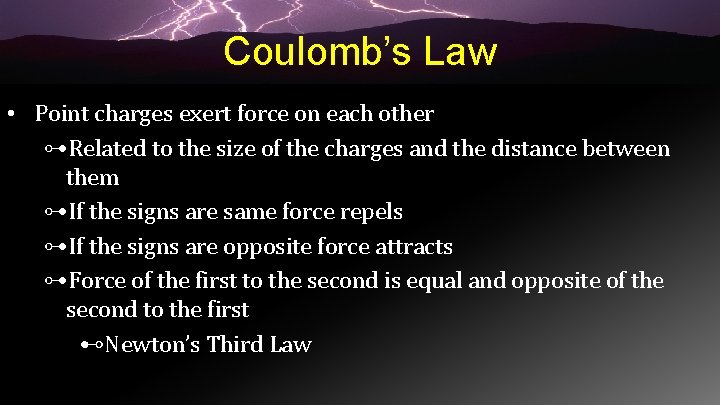Coulomb’s Law • Point charges exert force on each other ⊶Related to the size of the charges and the distance between them ⊶If the signs are same force repels ⊶If the signs are opposite force attracts ⊶Force of the first to the second is equal and opposite of the second to the first ⊷Newton’s Third LawCoulomb’s Law •Coulomb’s Law •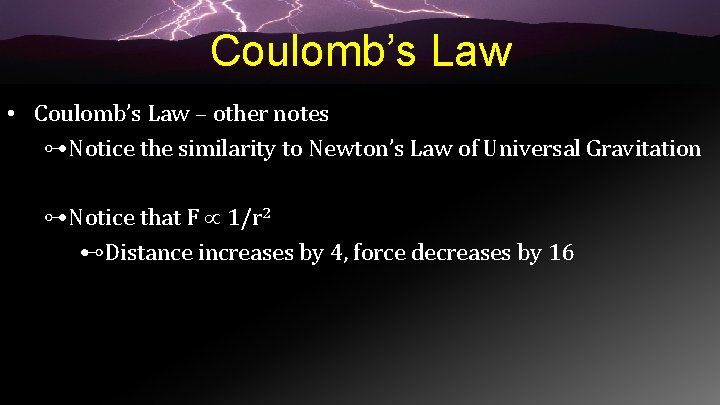Coulomb’s Law • Coulomb’s Law – other notes ⊶Notice the similarity to Newton’s Law of Universal Gravitation ⊶Notice that F 1/r 2 ⊷Distance increases by 4, force decreases by 16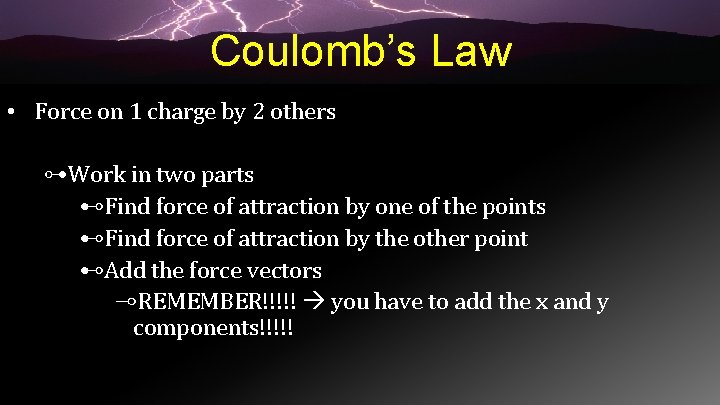Coulomb’s Law • Force on 1 charge by 2 others ⊶Work in two parts ⊷Find force of attraction by one of the points ⊷Find force of attraction by the other point ⊷Add the force vectors ⊸REMEMBER!!!!! you have to add the x and y components!!!!!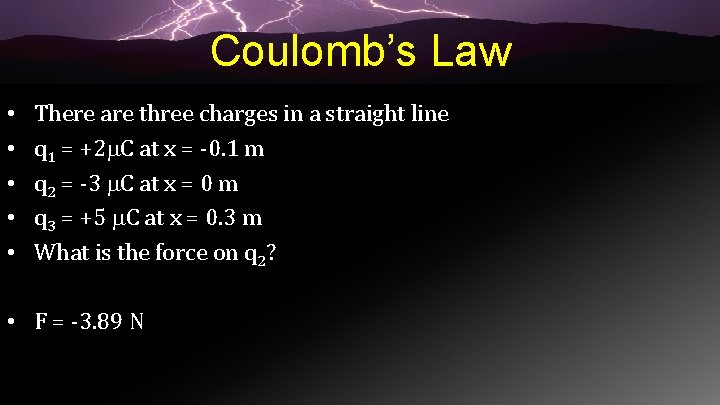Coulomb’s Law • • • There are three charges in a straight line q 1 = +2 C at x = -0. 1 m q 2 = -3 C at x = 0 m q 3 = +5 C at x = 0. 3 m What is the force on q 2? • F = -3. 89 N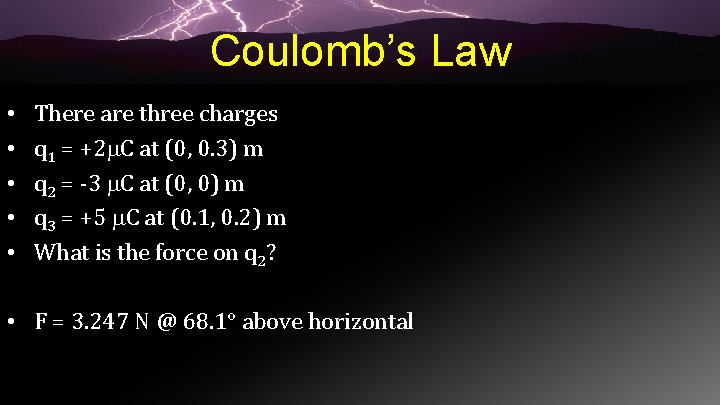Coulomb’s Law • • • There are three charges q 1 = +2 C at (0, 0. 3) m q 2 = -3 C at (0, 0) m q 3 = +5 C at (0. 1, 0. 2) m What is the force on q 2? • F = 3. 247 N @ 68. 1° above horizontalElectric Field and Electric Field Lines • We can use a test charge to determine how the surrounding charges generate a force • Pick a small test charge so it doesn’t change the surrounding charge orientation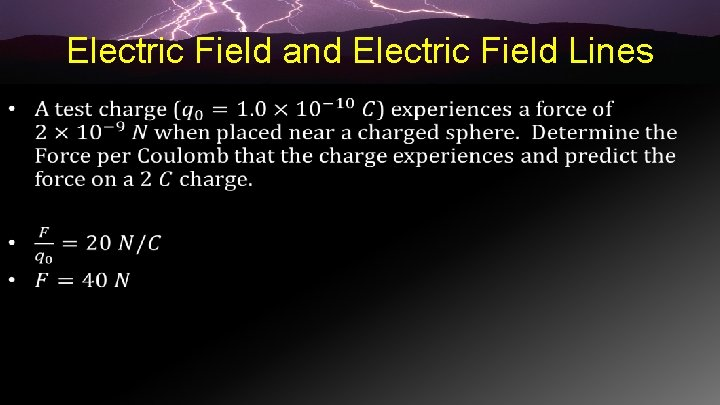Electric Field and Electric Field Lines •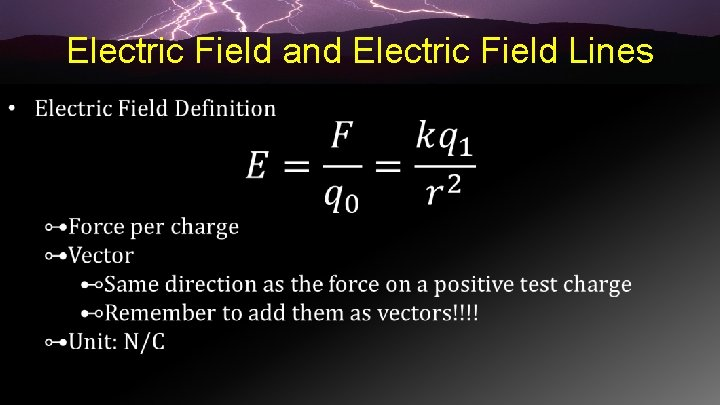Electric Field and Electric Field Lines •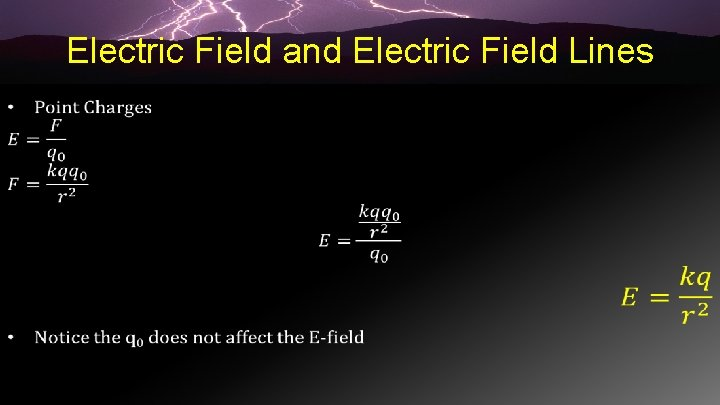Electric Field and Electric Field Lines •Electric Field and Electric Field Lines •Electric Field and Electric Field Lines • There are two point charges of q 1 = 4 C and q 2 = 8 C and they are 10 m apart. Find point where E = 0 between them. • d = 5. 85 m from q 2 towards q 1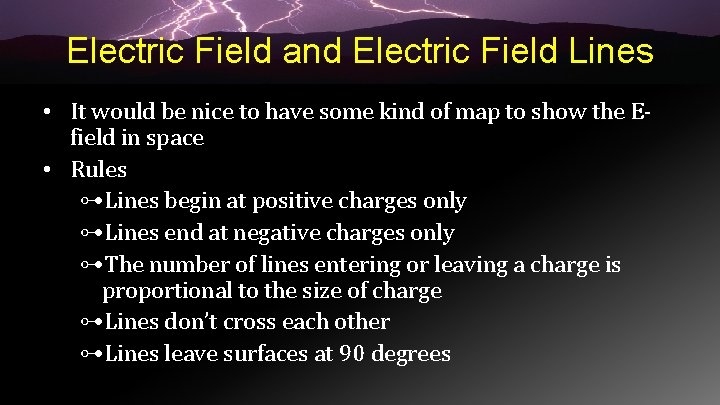Electric Field and Electric Field Lines • It would be nice to have some kind of map to show the Efield in space • Rules ⊶Lines begin at positive charges only ⊶Lines end at negative charges only ⊶The number of lines entering or leaving a charge is proportional to the size of charge ⊶Lines don’t cross each other ⊶Lines leave surfaces at 90 degrees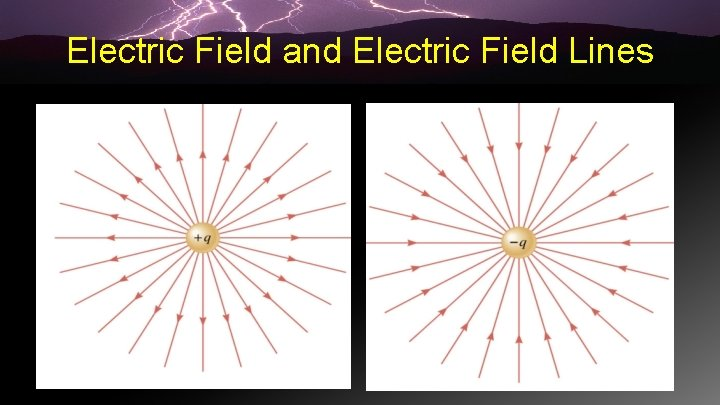Electric Field and Electric Field LinesElectric Field and Electric Field Lines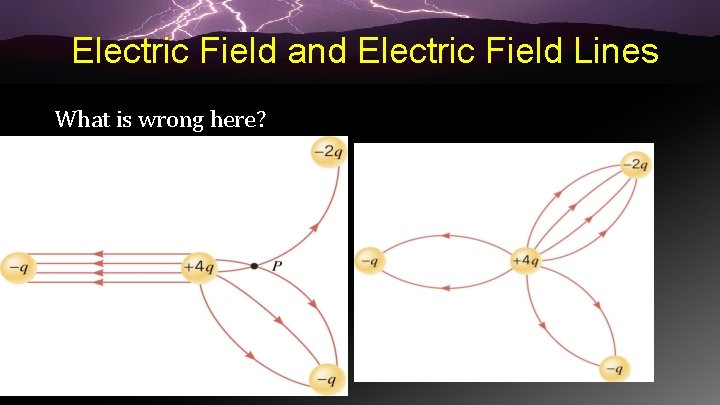Electric Field and Electric Field Lines What is wrong here?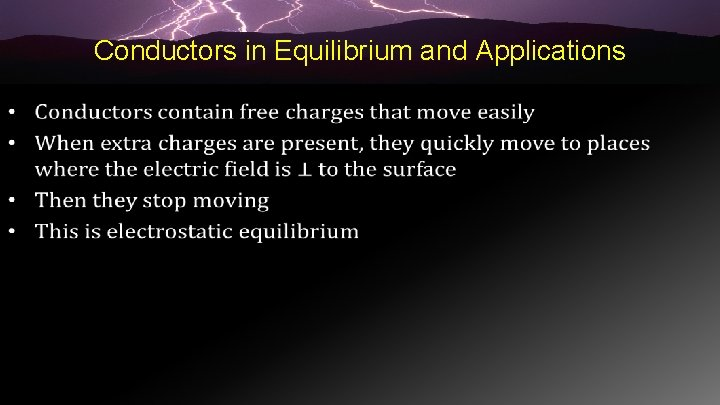Conductors in Equilibrium and Applications •Conductors in Equilibrium and Applications •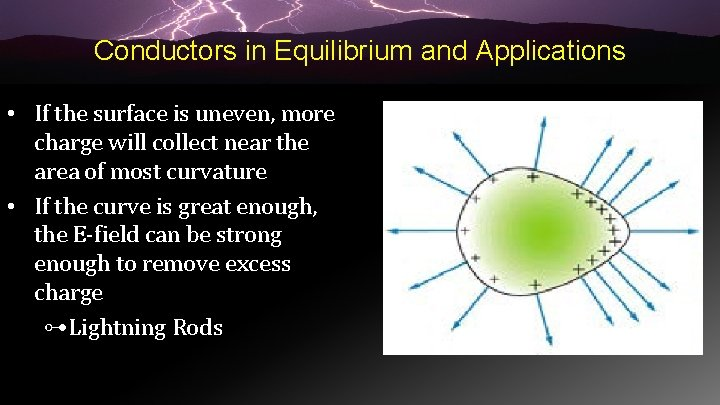Conductors in Equilibrium and Applications • If the surface is uneven, more charge will collect near the area of most curvature • If the curve is great enough, the E-field can be strong enough to remove excess charge ⊶Lightning RodsConductors in Equilibrium and Applications • Shielding ⊶A conductor shields any charge within it from external electrical fields ⊶Sensitive electrical equipment is shielded by putting in a metal box ⊷Called Faraday Cage ⊶Coaxial cable is shielded by a metal cylinder around the central metal wire. This reduces interference and signal loss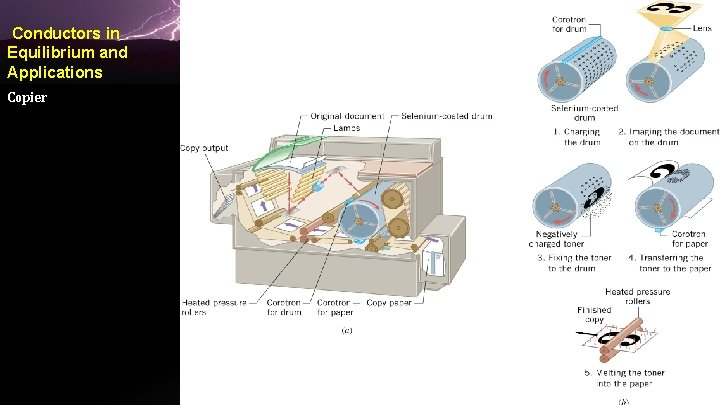Conductors in Equilibrium and Applications Copier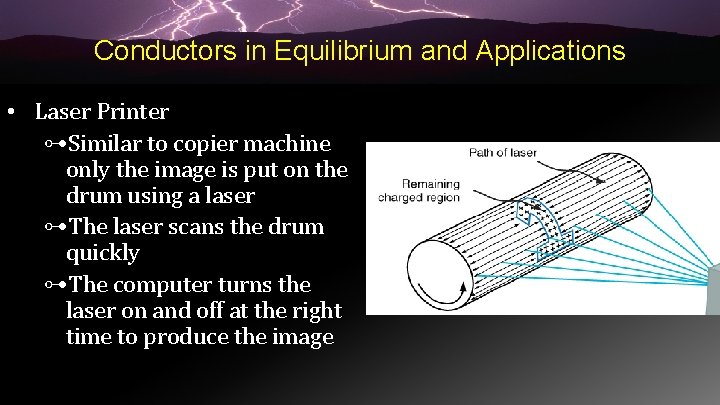Conductors in Equilibrium and Applications • Laser Printer ⊶Similar to copier machine only the image is put on the drum using a laser ⊶The laser scans the drum quickly ⊶The computer turns the laser on and off at the right time to produce the image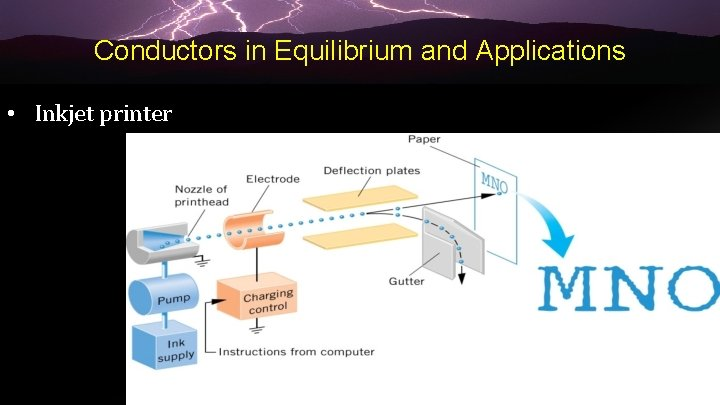Conductors in Equilibrium and Applications • Inkjet printer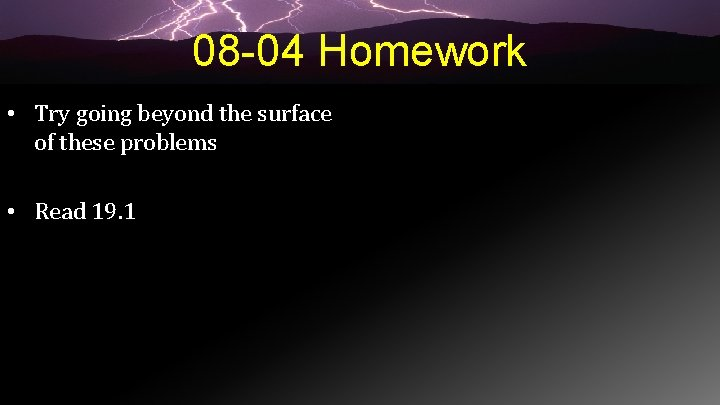08 -04 Homework • Try going beyond the surface of these problems • Read 19. 1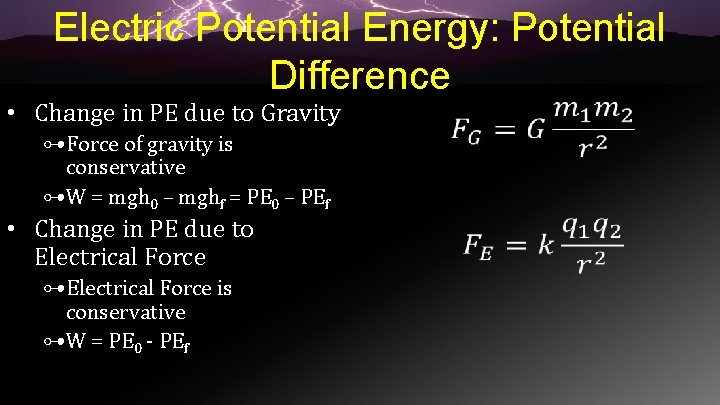Electric Potential Energy: Potential Difference • Change in PE due to Gravity ⊶Force of gravity is conservative ⊶W = mgh 0 – mghf = PE 0 – PEf • Change in PE due to Electrical Force ⊶Electrical Force is conservative ⊶W = PE 0 - PEf •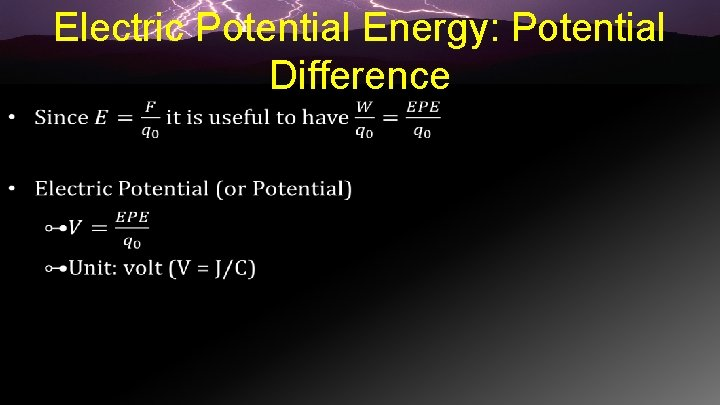Electric Potential Energy: Potential Difference •Electric Potential Energy: Potential Difference •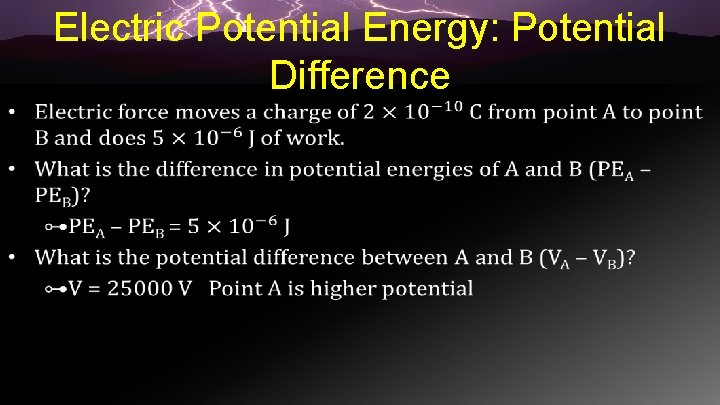Electric Potential Energy: Potential Difference •Electric Potential Energy: Potential Difference • Electric Potential Difference and Charge Sign ⊶Positive Charge ⊷Moves from higher electrical potential toward lower electrical potential ⊶Negative Charge ⊷Moves from lower to higher electrical potentials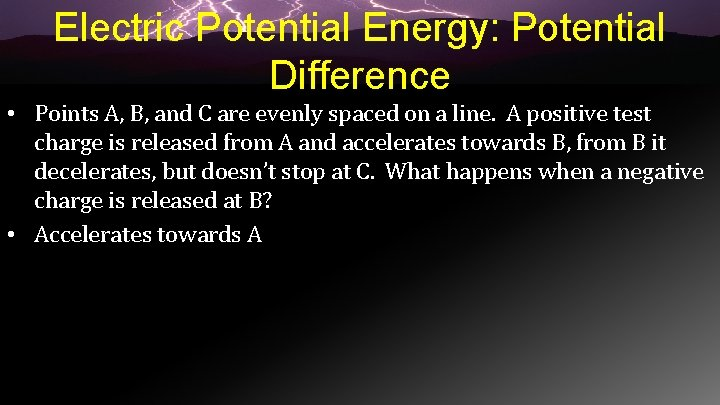Electric Potential Energy: Potential Difference • Points A, B, and C are evenly spaced on a line. A positive test charge is released from A and accelerates towards B, from B it decelerates, but doesn’t stop at C. What happens when a negative charge is released at B? • Accelerates towards A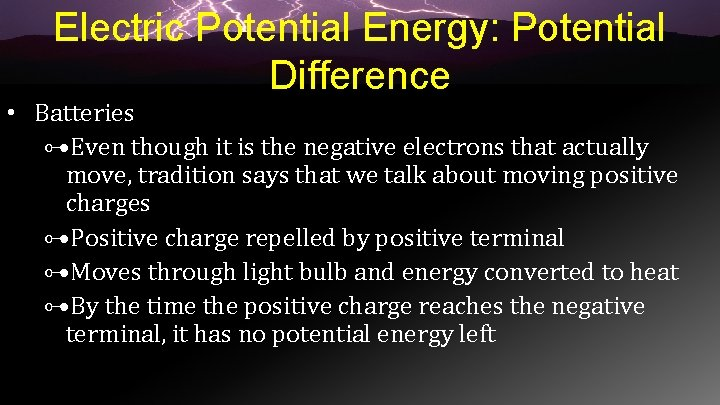Electric Potential Energy: Potential Difference • Batteries ⊶Even though it is the negative electrons that actually move, tradition says that we talk about moving positive charges ⊶Positive charge repelled by positive terminal ⊶Moves through light bulb and energy converted to heat ⊶By the time the positive charge reaches the negative terminal, it has no potential energy left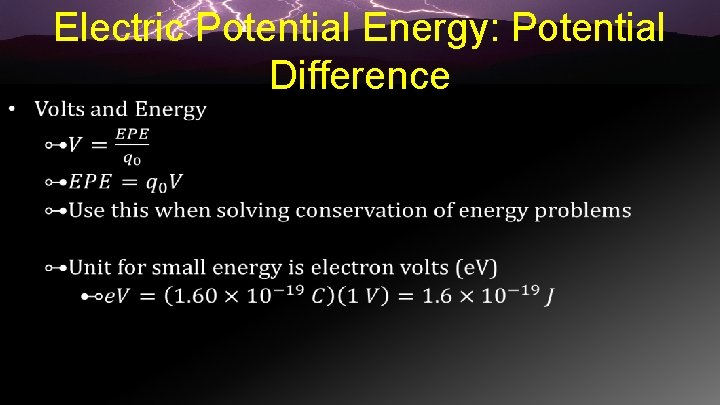Electric Potential Energy: Potential Difference •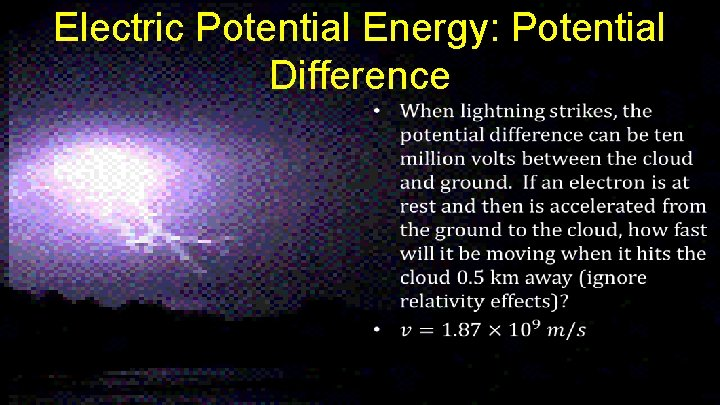Electric Potential Energy: Potential Difference •Electric Potential in a Uniform Electric Field • Both electric field and electric potential can be used to describe charges • E ⊶deals with force ⊶vector • V ⊶deals with energy ⊶scalarElectric Potential in a Uniform Electric Field •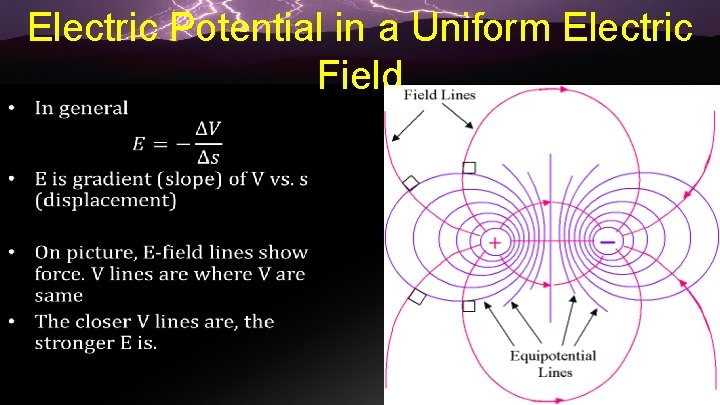Electric Potential in a Uniform Electric Field •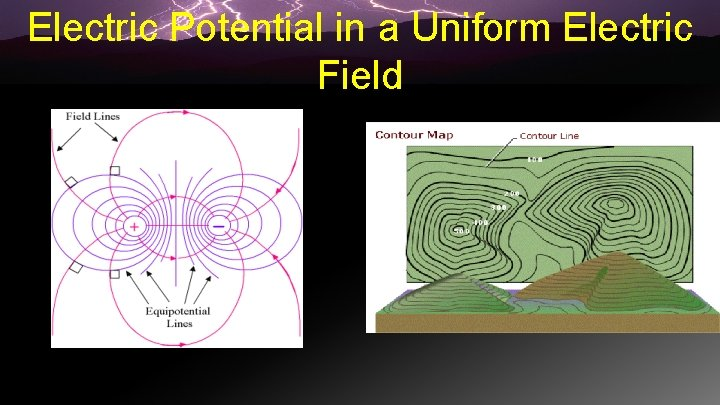Electric Potential in a Uniform Electric Field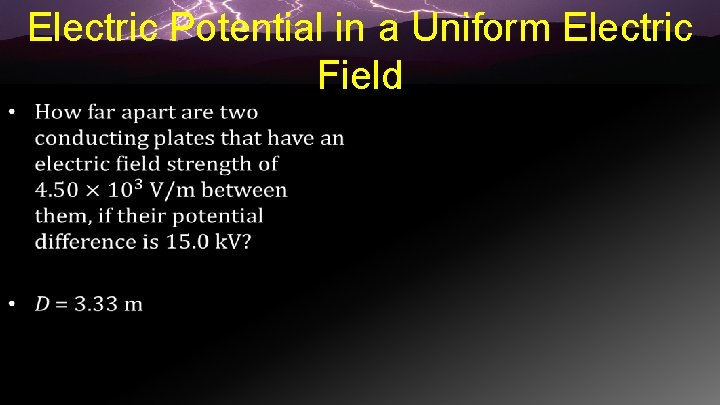Electric Potential in a Uniform Electric Field •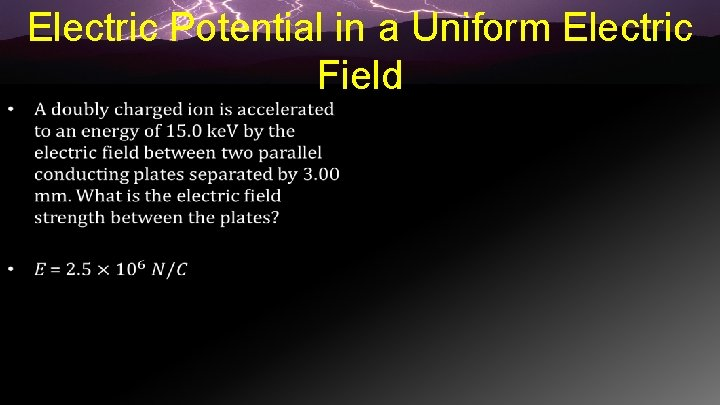Electric Potential in a Uniform Electric Field •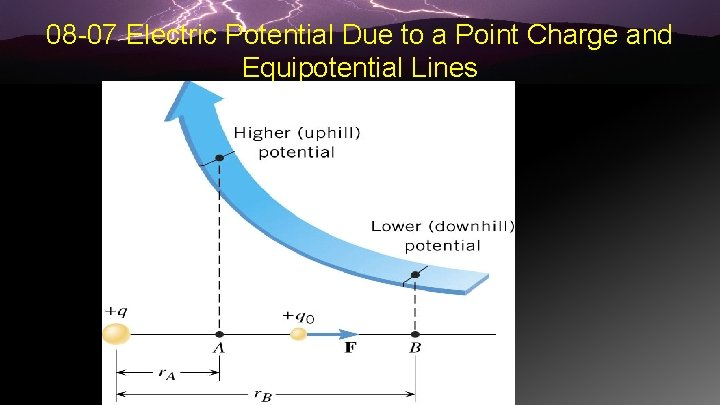08 -07 Electric Potential Due to a Point Charge and Equipotential Lines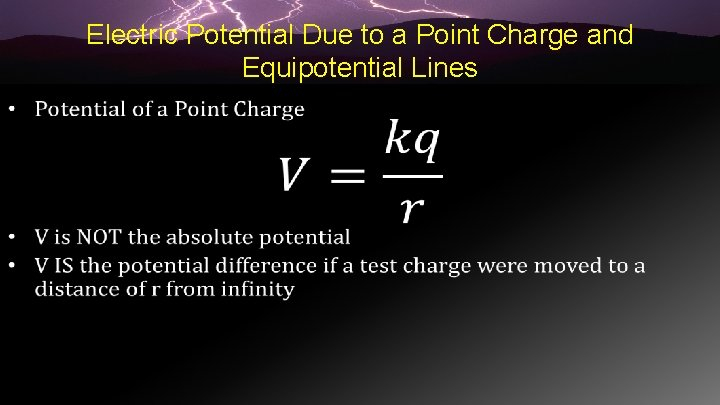Electric Potential Due to a Point Charge and Equipotential Lines •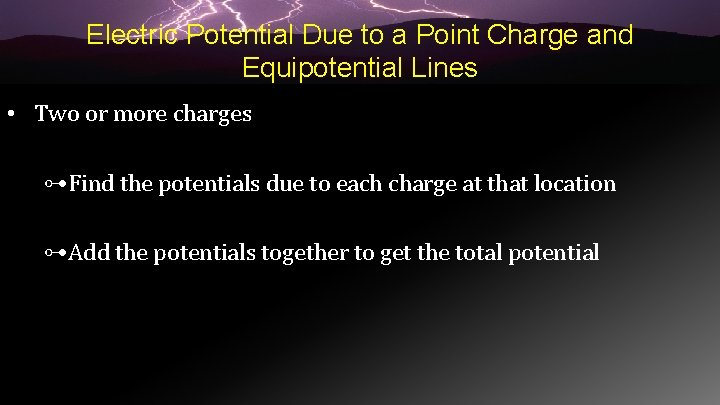Electric Potential Due to a Point Charge and Equipotential Lines • Two or more charges ⊶Find the potentials due to each charge at that location ⊶Add the potentials together to get the total potential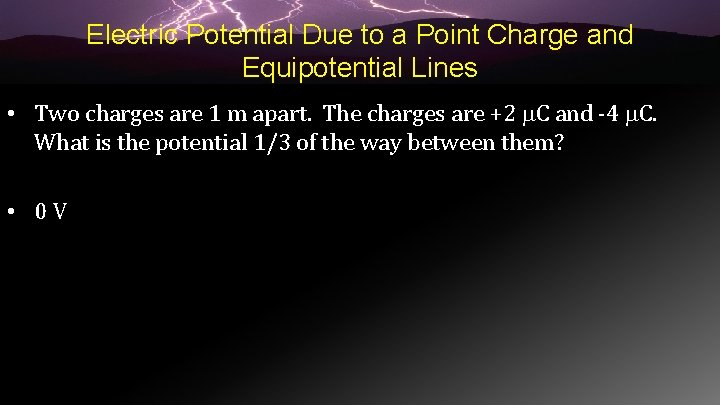Electric Potential Due to a Point Charge and Equipotential Lines • Two charges are 1 m apart. The charges are +2 C and -4 C. What is the potential 1/3 of the way between them? • 0 V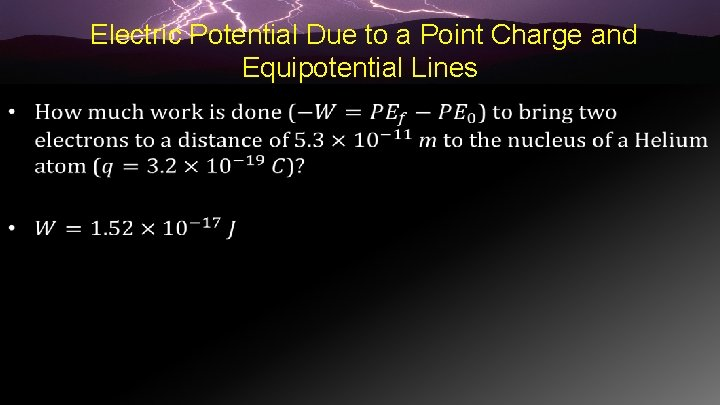Electric Potential Due to a Point Charge and Equipotential Lines •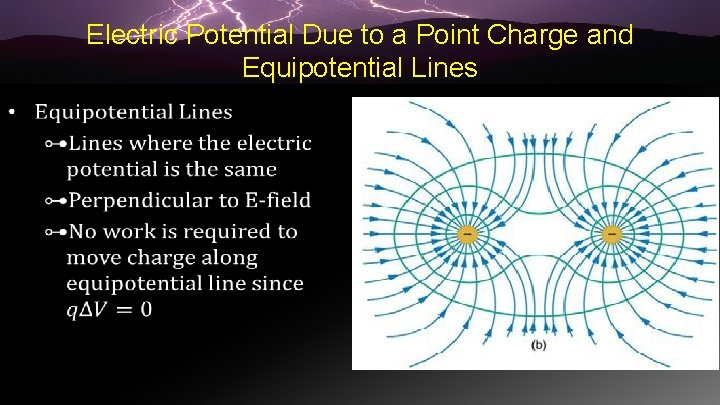Electric Potential Due to a Point Charge and Equipotential Lines •Electric Potential Due to a Point Charge and Equipotential LinesElectric Potential Due to a Point Charge and Equipotential Lines • Sketch the equipotential lines in the vicinity of two opposite charges, where the negative charge is three times as great in magnitude as the positive.Capacitors and Energy Stored in Capacitors • Capacitor ⊶Two parallel conductor plates separated by a gap ⊶Stores charge ⊷One plate +, one plate − ⊷Charge (Q) is the positive plateCapacitors and Energy Stored in Capacitors • Amount of charge stored depends on ⊶ Electric potential ⊶ Physical characteristic of capacitor (like distance) • Q = CV ⊶ Q = charge ⊶ C = capacitance ⊶ V = electric potential •Capacitors and Energy Stored in Capacitors • Some times the potential can • become so high that the air between the plates breaks down and starts conducting • To have higher capacitance, use some insulator in place of the air between the plates that doesn’t break down as easily • This is called dielectricCapacitors and Energy Stored in Capacitors •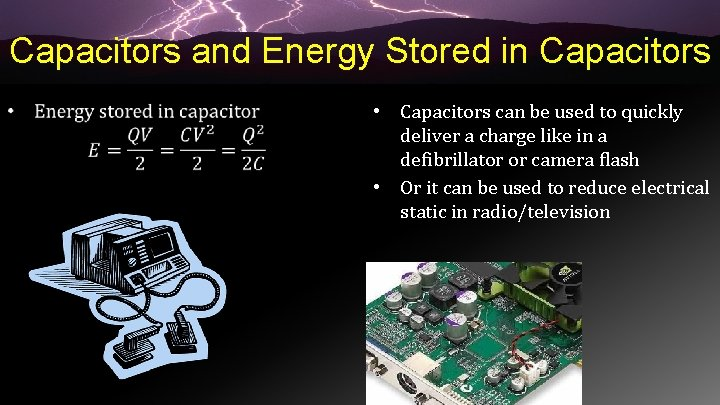Capacitors and Energy Stored in Capacitors • • Capacitors can be used to quickly deliver a charge like in a defibrillator or camera flash • Or it can be used to reduce electrical static in radio/televisionCapacitors and Energy Stored in Capacitors •